## PBMC 3k Analysis

scanpy process-test
In :
# Many fantastic pieces of free and open-source software can be used as key components to enable single cell analysis
# using python notebook. This scripts showed downstream analysis of 10X Genomic v3 RNAseq samples and import result
# into MongoDB for single cell explorer. The analytic codes were modified from scanpy tutorial "Clustering 3K PBMCs". We
#gratefully acknowledge all authors for Suerat and Scanpy and their contribution.
# we use 10K healthy donor's PBMCs data obtained from 10x Genomics, data can be downloaed from
# https://support.10xgenomics.com/single-cell-gene-expression/datasets/3.0.0/pbmc_10k_protein_v3

In [ ]:
# Creat data folder and download demo data from 10X genomics
!mkdir data
!wget http://cf.10xgenomics.com/samples/cell-exp/3.0.0/pbmc_10k_v3/pbmc_10k_v3_filtered_feature_bc_matrix.tar.gz -O data/pbmc_10k_v3_filtered_feature_bc_matrix.tar.gz
!cd data; tar -xzf pbmc_10k_v3_filtered_feature_bc_matrix.tar.gz

In :
import scpipeline

In :
import os,sys,csv,json,datetime,time,math,scipy.stats,collections,re;
from sklearn import preprocessing;
import numpy as np;
import pandas as pd;
import os.path;
import scanpy;
import scanpy.api as sc
sc.settings.set_figure_params(dpi=80)

In :
# read counts data from 10X V3 data (cell ranger V3 output)
p =  scpipeline.ProcessPipline();
dataPath='./data/filtered_feature_bc_matrix/';  # the directory with the .mtx file
p.data                                          # p.data: this is the data object we will use for QC

--> This might be very slow. Consider passing cache=True, which enables much faster reading from a cache file.
filtered out 10502 genes that are detected in less than 1 cells

Out:
AnnData object with n_obs × n_vars = 11769 × 23036
obs: 'n_genes', 'percent_mito', 'n_counts'
var: 'gene_ids', 'n_cells'
In :
### p.data is the data object for use
sc.pl.highest_expr_genes(p.data, n_top=10)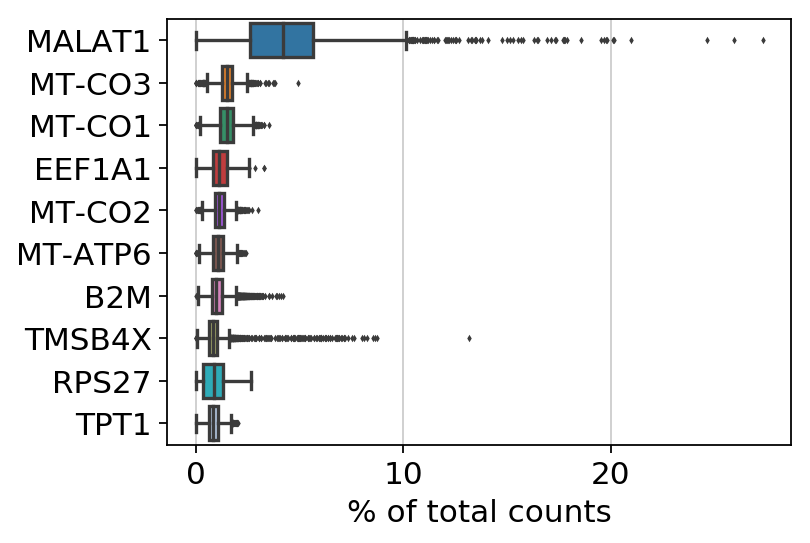In :
# QC function
# def QC(self,max_n_genes="" ,min_n_genes="",min_n_cells="",max_percent_mito="")
# scanpy tutorial QC(self,max_n_genes=2500 ,min_n_genes=200,min_n_cells=3,max_percent_mito=0.05)

p.QC(min_n_genes=200,min_n_cells=3)

filter cells
filtered out 232 cells that have less than 200 genes expressed
filter genes
filtered out 2684 genes that are detected in less than 3 counts

In :
## plot percentage of mitochondria
sc.pl.violin(p.data, ['n_genes', 'n_counts', 'percent_mito'],
jitter=0.4, multi_panel=True)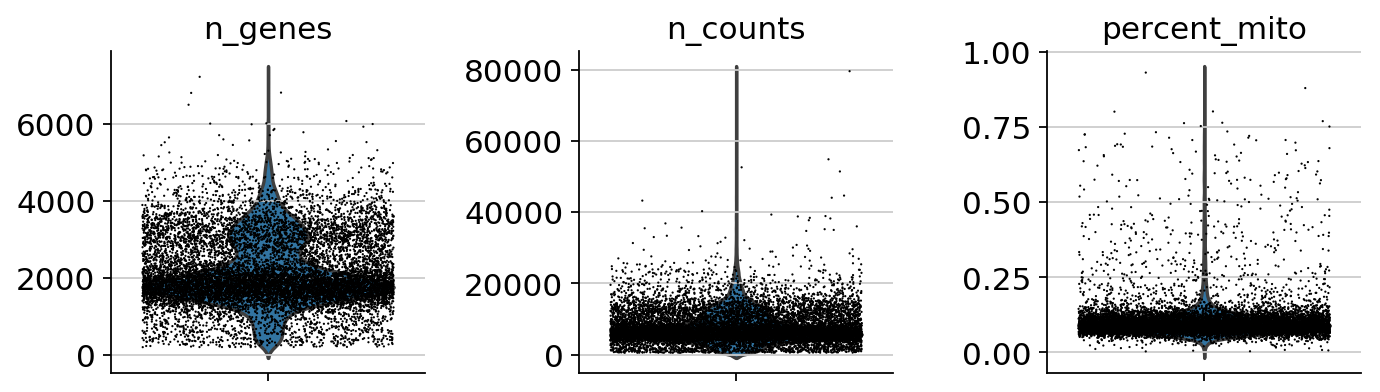In :
sc.pl.scatter(p.data, x='n_counts', y='n_genes')
sc.pl.scatter(p.data, x='n_counts', y='percent_mito')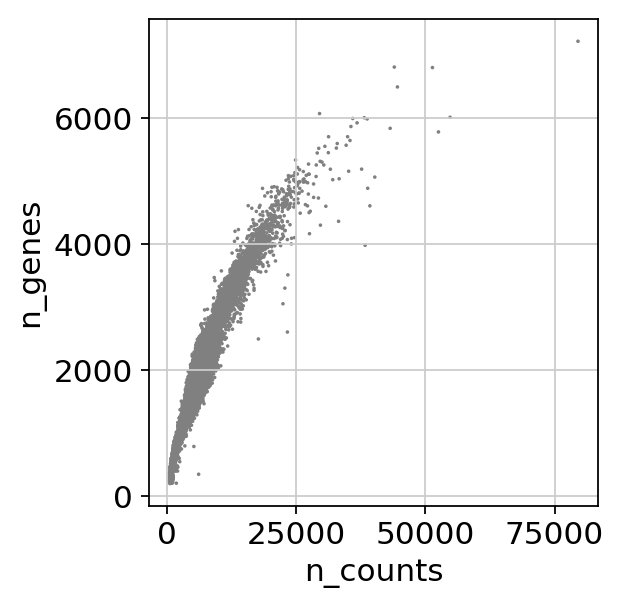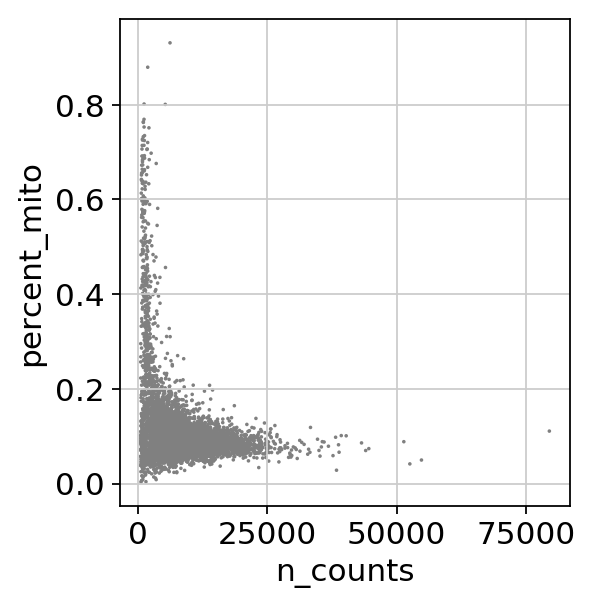In :
# QC using percentage of mitochondria gene
p.QC(max_n_genes=5000, max_percent_mito=0.12)
"""
# for those who are more famaliar with scanpy:
p.data = p.data[p.data.obs['n_genes'] < 5000, :]
p.data = p.data[p.data.obs['percent_mito'] < 0.12, :]
"""

filter n_genes < 5000
filter percent_mito < 0.12

Out:
"\n# for those who are more famaliar with scanpy: \np.data = p.data[p.data.obs['n_genes'] < 5000, :]\np.data = p.data[p.data.obs['percent_mito'] < 0.12, :]\n"
In :
# QC process in scanpy package will remove cell barcodes. However, for database loading, we adata should keep same number of barcodes as original one.
# We copy data from p.data to adata, which will be loaded into database


In :
adata

Out:
AnnData object with n_obs × n_vars = 9571 × 20352
obs: 'n_genes', 'percent_mito', 'n_counts'
var: 'gene_ids', 'n_cells', 'n_counts'
In :
# normalization (library-size correct) the data matrix to 10,000 reads per cell

In :
#Logarithmize the data

In :
adata.raw = adata

In :
# highly variable genes

--> added

In :
sc.pl.highly_variable_genes(adata)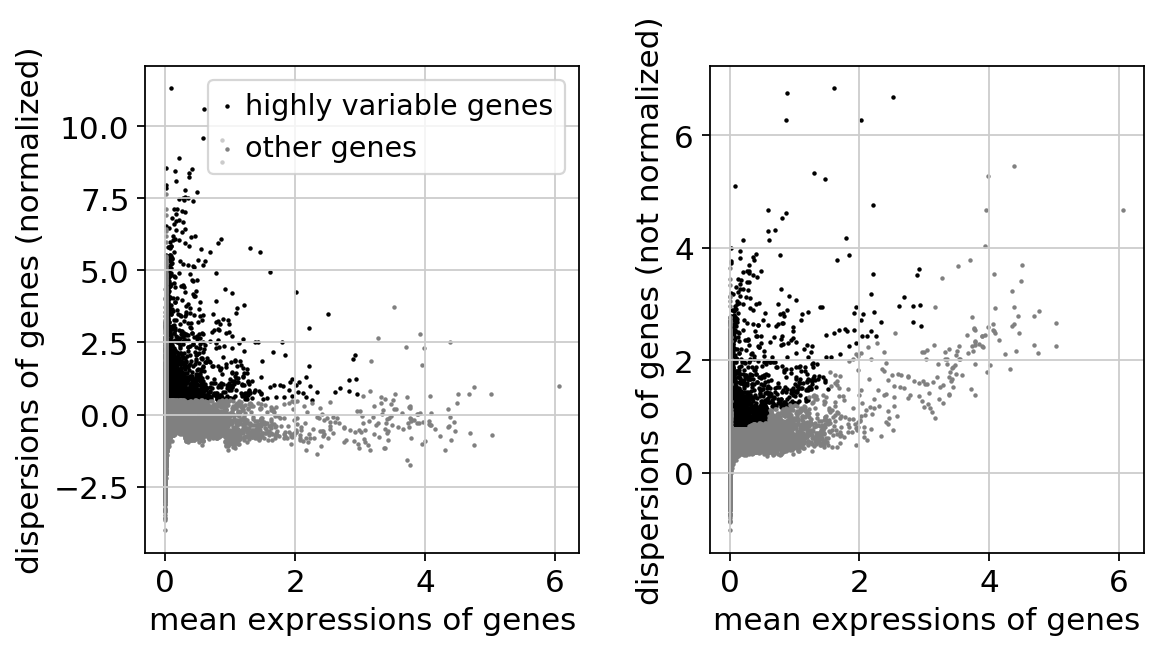In :
adata = adata[:, adata.var['highly_variable']]
# regress out effects of total counts per cell and the percentage of mitochondrial genes.
# Scale each gene to unit variance. Clip values exceeding standard deviation 10.

regressing out ['n_counts', 'percent_mito']
sparse input is densified and may lead to high memory use
finished (0:00:18.01)

In :
# Dimention reduction: PCA as a first step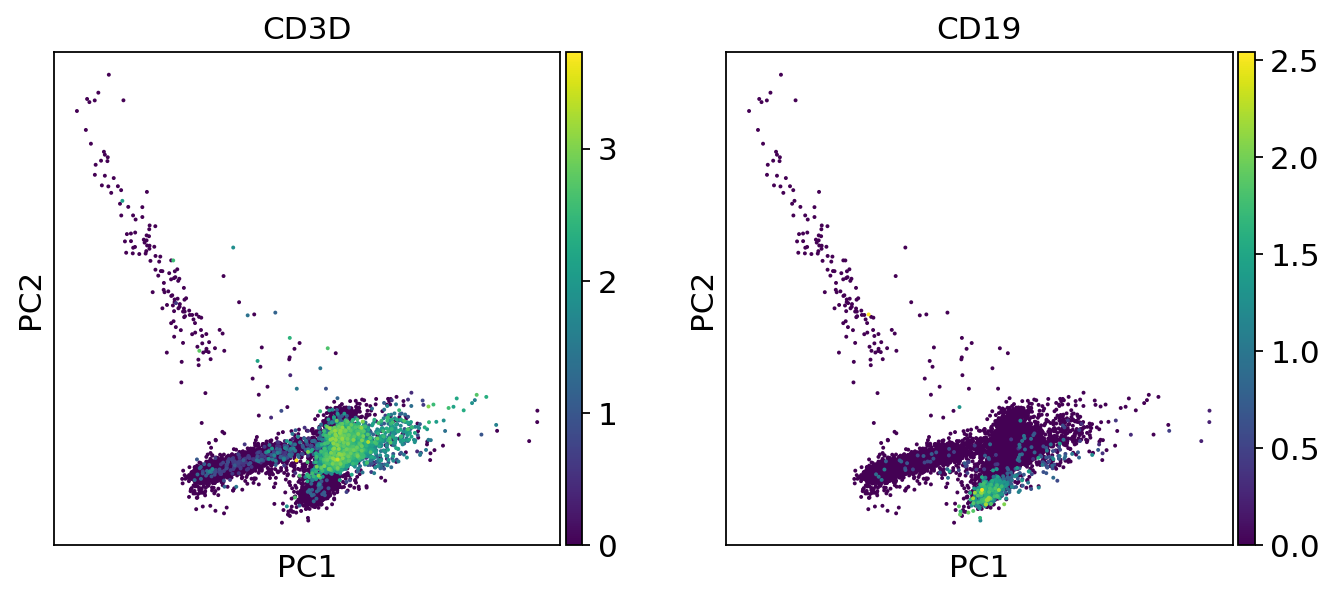In :
adata

Out:
AnnData object with n_obs × n_vars = 9571 × 2049
obs: 'n_genes', 'percent_mito', 'n_counts'
var: 'gene_ids', 'n_cells', 'n_counts', 'highly_variable', 'means', 'dispersions', 'dispersions_norm'
uns: 'pca'
obsm: 'X_pca'
varm: 'PCs'
In :
sc.pl.pca_variance_ratio(adata, log=True)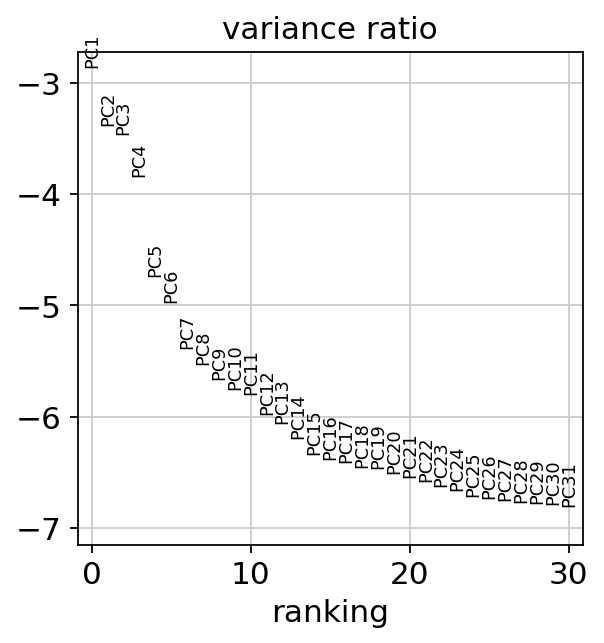In :
sc.pp.neighbors(adata, n_neighbors=10, n_pcs=40)

computing neighbors
using 'X_pca' with n_pcs = 40
finished (0:00:09.69) --> added to .uns['neighbors']
'distances', distances for each pair of neighbors

sc.tl.umap(adata)

computing UMAP

## check cel marker for T cell, B cells, Monocytes, and Dendritic Cells